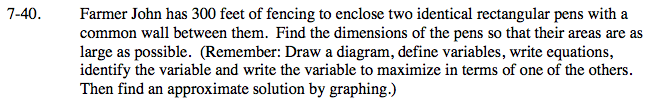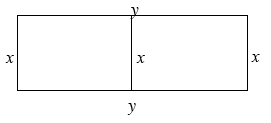### Home > PC > Chapter 7 > Lesson 7.2.1 > Problem7-40

7-40.1. Write one equation with the 300 feet of fencing for all of the sides.
2. Write another equation to maximize area: A = xy
3. Solve for one of the variables in the first equation and substitute in the second equation.
4. Use your calculator to find the place where the volume is the highest.